# Highlight duplicate values

In the previous post, we talked that how to highlight duplicate rows based on a certain number in excel. And this post will teach you how to highlight duplicate values in the range of cells in excel. Normally, you may be need to identify duplicate values with a range of cells in Excel. And there is one of the fasted way that is using conditional formatting feature in Microsoft Excel.

## Highlight duplicate values

You can easily identify duplicate values in a list, just do the following:

1# Select the range of cells that you want to check (or you can click one single cell firstly, then press shortcut CTRL +A)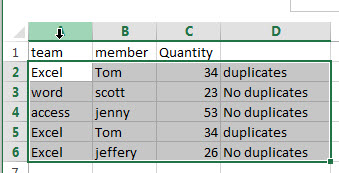2# On HOME Tab, click Conditional Formatting command in the styles group.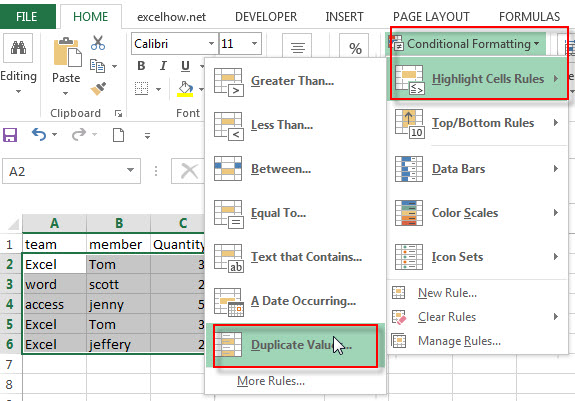3# click Highlight Cells Rules, then select Duplicate Values from the drop-down menu list.

4# select formatting styles, then click OK button to identify the duplicate values.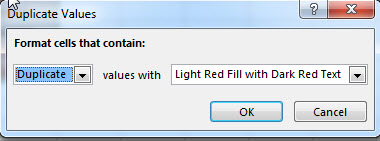5# you will see that the duplicate values in the range will now be highlighted.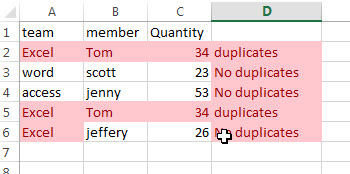## Highlight triplicate values

The above way will identify the duplicate values including duplicates, triplicates, or quadruplicates. If you only want to highlight triplicate values in your data, how to do it? At this time, you may be need to create your own formula to apply the conditional formatting.

Assuming that you want to highlight triplicate values in the range of cells A2:C4, then you can use the following formula based on the COUNTIF function:

`=COUNTIF(\$A\$2:\$C\$6,A2)=3`

This formula will check if the value of Cell A2 have three duplicates in the range A2:C4. If returns TRUE, then excel conditional formatting will highlight the color of cells.

Let’s see the below steps:

1# select the range of cells in your table

2# on the HOME tab, click the Conditional Formatting command under Styles group. Then select New Rules… from the drop-down menu list.

3# the New Formatting Rule window will appear.

4# select the Use a formula to determine which cells to format option under Select a Rule Type: box, and then enter the above formula in the Format values where this formula is true

5# click the Format… button, then the Format Cells window will appear.

6# in the “Format Cells” window, switch to the Fill tab, choose the background color, and then click OK button. You can also switch to other tabs to tweak the settings as you want.

7# back in the New Formatting Rule window, you can see a preview of your rows background color. Then click OK button.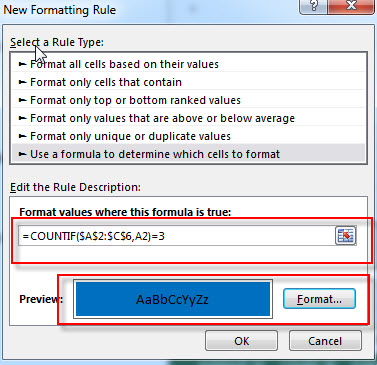8# let’s see the result: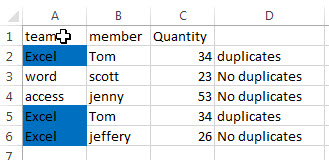If you want to highlight the duplicate values that is equal to the value of A2 in range A2:C7, and the duplicate values are more than 3 times, you can use the following formula as the conditional formatting rule.

`=COUNTIF(\$A\$2:\$C\$7,A2)>3`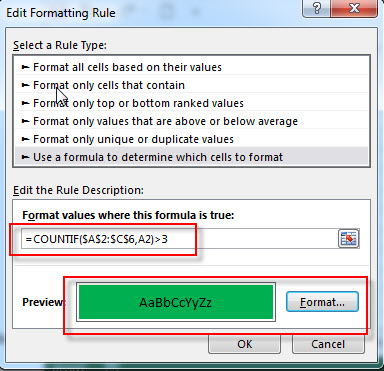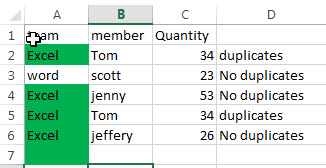### Related Functions

• Excel COUNTIF function
The Excel COUNTIF function will count the number of cells in a range that meet a given criteria. This function can be used to count the different kinds of cells with number, date, text values, blank, non-blanks, or containing specific characters.etc.= COUNTIF (range, criteria)…

### Related Posts

• Highlight Rows
You will learn that how to change the color of the entire rows if the value of cells in a specified column meets your conditions, such as, if the value of cells is equal to or greater than a certain number or text values, then excel should be highlight entire rows or change a row color as you need.…
• Find Duplicate Rows
If you want to check the entire row that duplicated or not, if True, then returns “duplicates” value, otherwise, returns “no duplicates”. You can create a formula based on the IF function and the SUMPRODUCT function..…
• Highlight Duplicate Rows
this post will talk that how to highlight entire rows that are duplicates in excel 2016, 2013 or lower version. Or how to change the background color of duplicate rows..…
• Combine Duplicate Rows and Sum the Values
This post will teach you how to combine duplicate rows and sum the corresponding values or calculate numbers in specific column in excel. And how to merge duplicate rows and then sum the values with VBA macro in Excel..…
Related Posts

Cap percentage values between 0 and 100

This article will talk about how to cap the percentage values between 0% and 100% in Microsoft Excel Spreadsheet or Google Sheets. If you are a newbie on Excel or google Sheets, you may be able to do this by ...

Calculate Cap Percentages to Specific Value

This article will talk about how to limit the cap percentage of a given amount to a given value in Microsoft Excel Spreadsheet or Google Sheets. If you are a newbie on Excel or google Sheets, you may be able ...

Calculate Win Loss Tie

Suppose you got a task to calculate the win, loss, and tie totals; what would you do? If you are new to Ms Excel and don't have enough experience with it, then you might do this task manually but let ...

Calculate Years Between Dates In Ms Excel

If you are an avid Ms Excel user, then you might have come across a task in which you needed to calculate the years between the dates; you might take it easy and do this task manually, which is also ...

Calculate Number of Hours between Two Times

Calculating the difference between two times might be a valuable statistic for subsequent computations or averages, whether you're producing a time sheet for staff or recording personal exercises. While Excel has a plethora of complex functions, including date and time ...

Calculate Loan Interest in Given Year

When you borrow money, you are supposed to repay it gradually. Lenders, on the other hand, want to be compensated for their services and the risk they incur by lending you money. That is, you will not just repay the ...

Calculate Interest Rate for Loan

The interest rate is the fee charged by a lender to a borrower and is expressed as a percentage of the principal—the lent amount. The interest rate on a loan is often expressed as an annual percentage rate, abbreviated as ...

Calculate Interest for Given Period

Using the IPMT function in Excel, we can compute the interest payment on any loan. This step-by-step tutorial will guide Excel users of all skill levels through the process to calculate interest for given period. Finally, the formula: =IPMT(B3/12,1,B5,-B2) The ...

How To Use Excel GCD Function

This post will guide you how to use Excel GCD function with syntax and examples in Microsoft excel. Description The Excel GCD function Returns the greatest common divisor of two or more integers. So you can use the GCD function ...

Calculate A Ratio From Two Numbers In Excel

In elementary mathematics, a ratio is a connection or comparison between two or more integers. For example, ratios are often expressed as ":" to demonstrate the relationship between two numbers. You would think that manually calculating a ratio from two ...

Sidebar